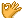•闪存
•博客
• 发言小组
• 投递新闻
• 提问博问
• 添加收藏
• 发布招聘
•文库

3

##10小白求问语法报错原因

row_input=int(input('请输入行数：') row=1 while row<=row_input: row_h=1 while row_h<=row: print('*',end=''